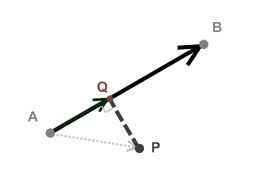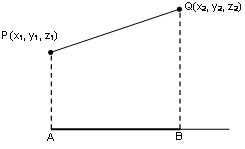Click to Chat

1800-1023-196

+91-120-4616500

CART 0

• 0

MY CART (5)

Use Coupon: CART20 and get 20% off on all online Study Material

ITEM
DETAILS
MRP
DISCOUNT
FINAL PRICE
Total Price: Rs.

There are no items in this cart.
Continue Shopping```Projection of a Line

What Exactly do We mean by the Projection of a Point on a Line?

Consider a point P and AB be the given line. Now construct a perpendicular PQ from P on AB which meets AB at Q. This point Q is termed as the projection of P on the line AB.What is the Projection of a Line Segment Joining two Points on a Line?

Let PQ be a line segment where P = (x1, y1, z1) and Q = (x2, y2, z2) and AB be a given line with dc’s as l, m, n. If the line segment PQ makes an angle θ with the line AB, then the projection of PQ is P’Q’ = PQ cos θ

Now, substituting the value of cos θ in this result, we obtain the following value of P’Q’

P’Q’ = l(x2-x1) + m(y2-y1) + n(z2-z1)Some Important Facts

We know that for x-axis, we have l = 1 and m = n = 0. Hence,

Projection of PQ on x- axis = 1(x2-x1) + 0(y2-y1) + 0(z2-z1) = (x2-x1)

Projection of PQ on y- axis = 0(x2-x1) + 1(y2-y1) + 0(z2-z1) = (y2-y1)

Projection of PQ on z- axis = 0(x2-x1) + 0(y2-y1) + 1(z2-z1) = (z2-z1)

We know that PQ2 = (x2-x1)2 + (y2-y1)2 + (z2-z1)2 which shows that

If a, b, c are the projections of a line segment on coordinate axis then the length of the segment = √(a2 + b2 + c2)

If a, b, c are the projections of a line segment on coordinate axis then its dc’s are ± a/√(a2 + b2 + c2), ± b/√(a2 + b2 + c2), ± c/√(a2 + b2 + c2)

Perpendicular Distance of a Point from a Line

Let AB be the straight line passing through the point A (a, b, c) and having direction cosines l, m, and n. Now, if AN is assumed to be the projection of line AP on the straight line AB then we have

AN = l(x – a) + m(y – b) + n(z – c)

and AP = √(x–a)2 + (y–b)2 + (z–c)2

∴ Perpendicular distance of point P

PN = √(AP2 – AN2)The length of the perpendicular from a point (x1, y1, z1) to a plane ax+ by + cz + d = 0 is

p = [(ax1 + by1 + cz1 + d)/ √(a2 + b2 + c2) ]

For more, view the following video

Illustration:

Find the perpendicular distance of point P (0, 2, 3) from straight line passing through A( 1, -3,2) given that the direction ratios are 1, 2, 2.

Solution:

We know that if we have the direction ratios, then we can easily compute the direction cosines.

If ‘a’, ‘b’, and ‘c’ are the direction ratios, then the direction cosines are given by

a/√(a2+b2+c2),  b/√(a2+b2+c2),  c/√(a2+b2+c2).

Here, we have the direction ratios as a = 1, b = 2, c = 2.

Hence, the direction cosines are 1/3, 2/3, 2/3.

Therefore, PN = l(x – a) + m(y – b) + n(z – c)

= 1/3 (0 – 1) + 2/3 (2 + 3) + 2/3 (3 – 2)

= -1/3 + 10/3 + 2/3

= 11/3

AP = √(0–1)2 + (2 + 3)2 + (3–2)2 = √27

∴ Perpendicular distance PN = √AP2 – PN2 = √27–121/9 = √122/3.

Related Resources

Look into the past year papers to get an idea about the types of questions asked in the exam.

Various Recommended Books of Mathematics are just a click away.

```### Course Features

• 731 Video Lectures
• Revision Notes
• Previous Year Papers
• Mind Map
• Study Planner
• NCERT Solutions
• Discussion Forum
• Test paper with Video Solution